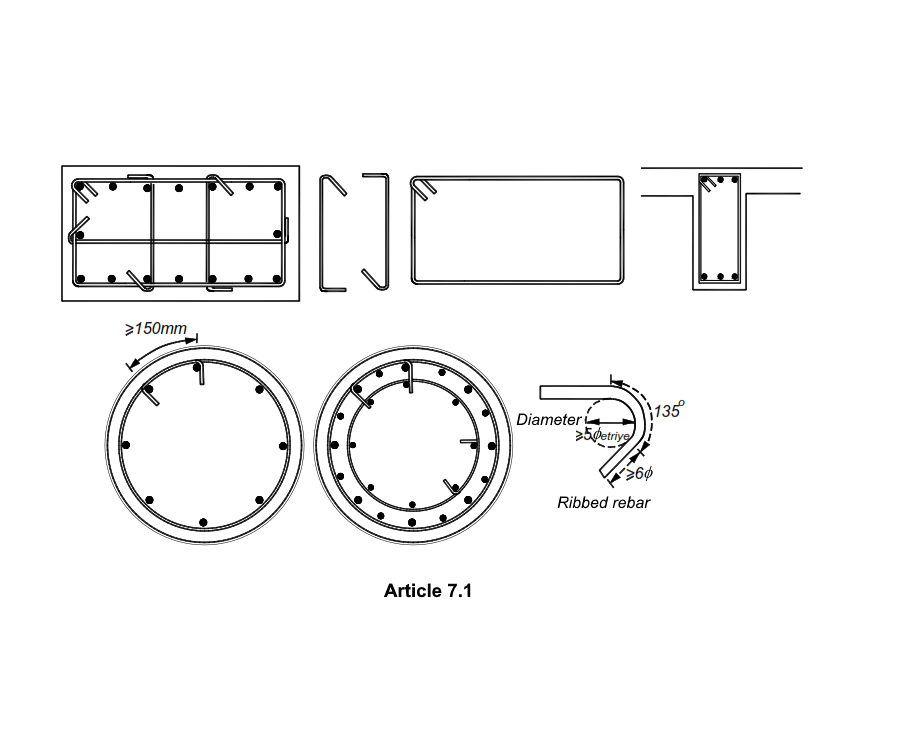# Shear Strength Controls of Columns

#### HakanŞahin7.3.7.1 - Shear force V e to be taken as basis for transverse reinforcement calculation in columns , Eq. It is calculated by (7.5) .

It is important for the calculation of M a and M ü whether the sum of the bearing strength moments of the columns at the node point is 20% more than the moments of the beams in both directions of the earthquake . For this reason, it is first checked whether the equation Equation (7.3) is fulfilled.

Click on the links below to examine in detail how the calculations are made.